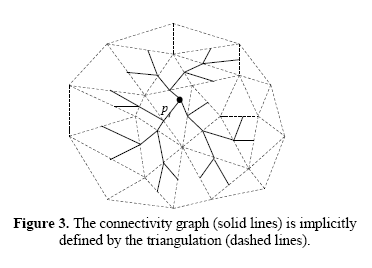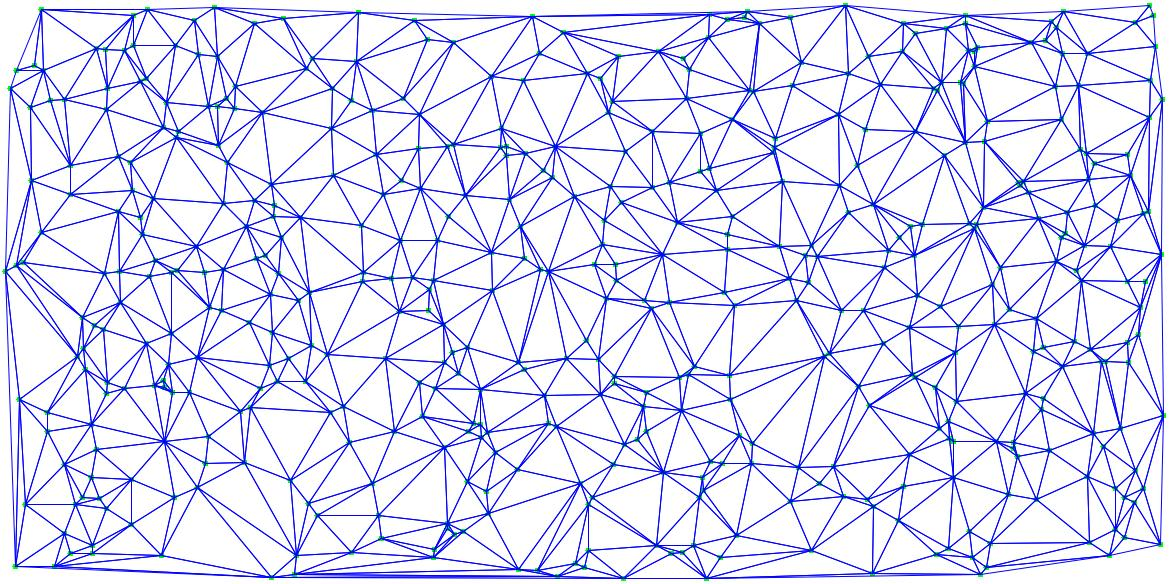2 views

I have an area that is represented by constrained Delaunay triangulations. I am playing around with the problem of finding a path between two points. I am using the paper provided by Marcelo Kallmann as a reference point to approach this problem. However, instead of using the Chazelle funnel algorithm as proposed by Kallmann paper link, I am planning to use the A* search algorithm which plans the path in a grid environment pretty efficiently.

For the heuristic cost function, I am using the Euclidean distance from the current node to the goal node and for selecting the neighbor nodes, I am drawing a line segment from the current point p to the midpoint of the edge of the triangle. [This idea was proposed by kallmann] The cost to a neighbor node is also given by the Euclidean distance among them.

Here is the figure from the Kallmann demonstrating the use of the mid-point of the edge technology to generate various paths to the triangle containing the goal node.Now, this technique works fine when the triangle density is not large enough in an area. But say if the generated triangulation for a set of points looks like thisI was wondering whether there is a way to improve the efficiency of the algorithm by using some other technique like IDA* or ID-DFS? A Triangulation Reduction A* (TRA*) has been proposed, but I could not understand it since it was too formally defined. The details of the TRA* method can be found in section 5 of this thesis by Demyan.

I would appreciate some thoughts on it. If some code is required to be shared, I will edit this post.

by (108k points)

Note that the ID algorithms trade off the cost of bookkeeping acquired by A* by repeating work, thus potentially making them slower (but hopefully not much slower). So whatever measure you're trying to improve the efficiency of will affect how useful these will be.

If you are looking to learn more about Artificial Intelligence then you visit Artificial Intelligence(AI) Tutorial. Also, if you are appearing for job profiles of AI Engineer or AI Expert then you can prepare for the interviews on Artificial Intelligence Interview Questions.